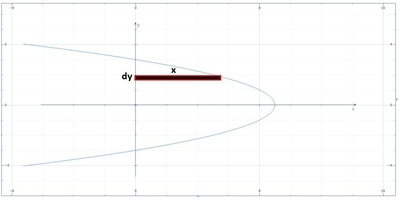# The base of a solid is the region bounded by the function x = -y^2 + 9 and the y-axis. Set up the...

## Question:

The base of a solid is the region bounded by the function {eq}x = -y^2 + 9 {/eq} and the {eq}y {/eq}-axis. Set up the integral that gives the volume of the solid if every cross section perpendicular to the {eq}y {/eq}-axis is a square.

## Volume of the Solid:

This problem is about solving the volume of the solid using the slicing method which uses the formula {eq}V=A\times l {/eq} where {eq}A {/eq} is the area of the cross-section and {eq}l {/eq} is the length.

## Answer and Explanation:

Below is the graph,From the graph the differential volume is,

{eq}dV= \left ( x^{2} \right )dy {/eq}

{eq}V= \int_{a}^{b}x^{2}dy {/eq}

{eq}V= \int_{a}^{b}\left ( -y^{2}+9 \right )^{2}dy {/eq}

For the limits, from the given function when {eq}x=0 {/eq}

{eq}0=-y^{2}+9 {/eq}

{eq}y=-3,3 {/eq}

Thus,

{eq}V= \int_{-3}^{3}\left ( -y^{2}+9 \right )^{2}dy {/eq}

Integrate,

{eq}V=\left [ \frac{y^5}{5}-6y^3+81y \right ]^{3}_{-3} {/eq}

{eq}V=\frac{1296}{5} {/eq}

{eq}V=259.2 {/eq}

#### Learn more about this topic:Volumes of Shapes: Definition & Examples

from GMAT Prep: Tutoring Solution

Chapter 11 / Lesson 9
45K